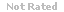Loading... Please wait...

(TCO D) Common Stock of Coquihalla Corporation will pay a dividend of \$8.00 in the upcoming year, and dividends are expected to grow 5% per year in the future.

Price:
\$15.99

Product Description

1. (TCO D)  Common Stock  of Coquihalla Corporation will pay a dividend of \$8.00 in the upcoming year, and dividends are expected to grow 5% per year in the future. Your  required rate of annual  return is 12% . Using the Constant  Dividend  Growth Model  calculate the current price of the Common Stock. (Points : 20)

2. An investor is looking to buy a bond that currently pays \$155 a year in interest (coupon rate). The current yield is 11% and the face value is \$1,000.  How much will the investor have to pay for this bond? (Points : 20)

3. TCO G)  An investor purchases 300 shares of ABC stock for \$15.00 a share and immediately sells 2 covered call contracts at a strike price of \$20.00 a share. The premium is \$2.00 a share.  What is the maximum profit and the maximum loss?

4. (TCO E)  Identify two limitations of technical analysis. (Points : 20)

5. (TCO A)  Identify the three levels of subscribers to the NASDAQ. (Points : 20)

6. (TCO B)  There are 3 versions of the Efficient Market Hypothesis. Briefly describe each. (Points : 20)

7. (TCO F) You are an analyst comparing the performance of two portfolio managers using the Sharpe Ratio measurement. Manager A shows a return of 20% with a standard deviation of 17%. Manager B shows a return of 13% with a standard deviation of 6%. If the risk free rate is 5% which manager has the better risk adjusted return? (Points : 20)

8. (TCO I)  Look at the following Balance Sheet and calculate the following ratios:  current ratio, quick ratio, and the  debt ratio.

9. (TCO J)  What are the two primary disadvantages of investing internationally? (Points : 20)

10. (TCO H)  Why was "Limited-Time Shelf Registration" introduced as a regulation? (Points : 20)

11. (TCO D) Diversification in an investment portfolio is a significant concept for creating the highest return for the least amount of risk. To create this diversification portfolio managers consider the covariance and correlation of investments. Explain how covariance and correlation help to create this diversification. (Points : 25)

Customers Who Viewed This Product Also Viewed

• \$8.99
• \$19.99
• \$8.99
• \$22.99
• \$8.99

• \$15.99